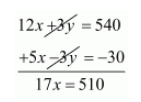# In a ∆ABC, ∠A = x°, ∠B = 3x° and ∠C = y°. If 3y − 5x = 30,`
Question:

In a $\triangle A B C, \angle A=x^{\circ}, \angle B=3 x^{\circ}$ and $\angle C=y^{\circ}$. If $3 y-5 x=30$, prove that the triangle is right angled.

Solution:

We have to prove that the triangle is right

Given $\angle A=x^{\circ}, \angle B=3 x^{\circ}$ and $\angle C=y^{\circ}$

Sum of three angles in triangle are $\angle A+\angle B+\angle c=180^{\circ}$

$\angle A+\angle B+\angle c=180^{\circ}$

$x+3 x+y=180$

$4 x+y=180 \cdots(i)$

By solving $4 x+y=180$ with $3 y-5 x=30$ we get,

$4 x+y=180$

$-5 x+3 y=30 \cdots(i i)$

Multiplying equationby 3 we get

$12 x+3 y=540 \cdots($ iii $)$

Subtracting equation (ii) from (iii)$x=\frac{510}{17}$

$x=30^{\circ}$

Substituting $x=30^{\circ}$ in equation $(i)$ we get

$4 x+y=180$

$4 \times 30+y=180$

$120+y=180$

$y=180-120$

$y=60^{\circ}$

Angles $\angle A, \angle B$ and $\angle C$ are

$\angle A=x^{\circ}$

$=30^{\circ}$

$\angle B=3 x^{\circ}$

$=3 \times 30^{\circ}$

$=90^{\circ}$

$\angle C=y^{\circ}$

$=60^{\circ}$

A right angled triangle is a triangle in which one side should has a right angle that is $90^{\circ}$ in it.

Hence, $\angle B=90^{\circ}$ The triangle $\mathrm{ABC}$ is right angled